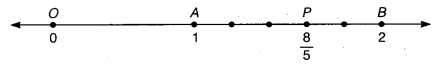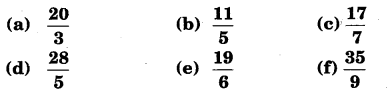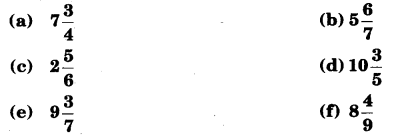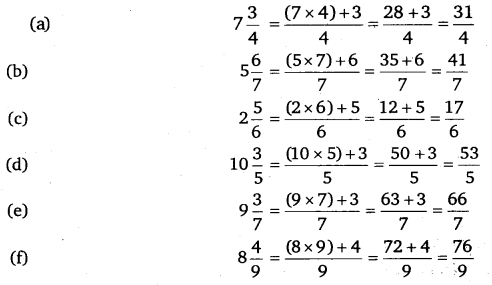# Class 6 Maths NCERT Solutions for Chapter 7 Fractions Ex – 7.2

## Fractions

Question 1.
Draw number lines and locate the points on them:

(a)$\frac { 1 }{ 2 } ,\frac { 1 }{ 4 } ,\frac { 3 }{ 4 } ,\frac { 4 }{ 4 }$
(b)$\frac { 1 }{ 8 } ,\frac { 2 }{ 8 } ,\frac { 3 }{ 8 } ,\frac { 7 }{ 8 }$
(c)$\frac { 2 }{ 5 } ,\frac { 3 }{ 5 } ,\frac { 8 }{ 5 } ,\frac { 4 }{ 5 }$

Solution:
(a)
We know that$\frac { 1 }{ 2 }$ is greater than zero and less than 1, so it should lie 2 between 0 and 1.
To show$\frac { 1 }{ 2 }$ on the number line, we divide the gap between 0 and 1 into two equal parts and the point P represents the fraction$\frac { 1 }{ 2 }$ .To represent$\frac { 1 }{ 4 } ,\frac { 3 }{ 4 } ,\frac { 4 }{ 4 }$ on the number line, we divide the gap between 0 and 1 into 4 equal parts, then the points P, R and A respectively represent the fraction$\frac { 1 }{ 4 } ,\frac { 3 }{ 4 }$ and$\frac { 4 }{ 4 }$ .

(b)
To rfepresent$\frac { 1 }{ 8 } ,\frac { 2 }{ 8 } ,\frac { 3 }{ 8 }$ and$\frac { 7 }{ 8 }$ on the number line, we divide the gap between 0 and 1 into 8 equal parts, then the points P, Q, R and S respectively represent the fraction$\frac { 1 }{ 8 } ,\frac { 2 }{ 8 } ,\frac { 3 }{ 8 }$ and$\frac { 7 }{ 8 }$ .

(c)
To represent$\frac { 2 }{ 5 } ,\frac { 3 }{ 5 }$ and$\frac { 4 }{ 5 }$ on the number line, we divide the gap between 0 and 1 into 5 equal parts, then the points P, Q and R respectively represent the fraction$\frac { 2 }{ 5 } ,\frac { 3 }{ 5 }$ and$\frac { 4 }{ 5 }$ .For representing$\frac { 8 }{ 5 }$
Draw a line. Take a point 0 on it. Let it represent 0.
Now,$\frac { 8 }{ 5 } =1\quad \frac { 3 }{ 5 } =1+\frac { 3 }{ 5 }$From O, set off unit distances to the right. Let these segments be OA, AB. Then, clearly the points A and B represent 1 and 2 respectively. Take 1 full unit length to the right of O. Divide the 2nd unit AB into 5 equal parts. Take 3 parts out of these 5 parts to reach P. Then, P represents$\frac { 8 }{ 5 }$.

Question 2.
Express the following as mixed fractions:Solution:Question 3.
Express the following as improper fractions:Solution: